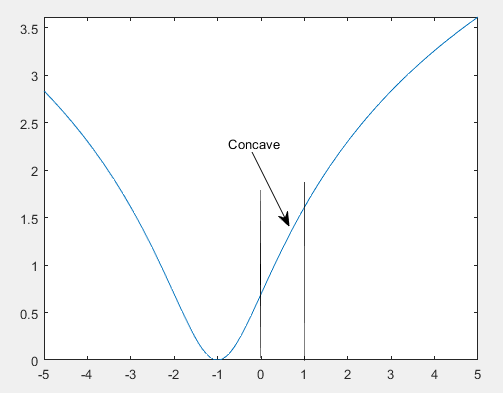# How to handel log convex

Hello everyone!

How can I write log(1+(1+x)^2) in CVX?

Thanks!

does not seem neither convex nor concave. See

However, I have the following constraint : 0<=x<=1.
According to the MATLAB plot, this function is nearly concave in this interval!

So, this constraint can make my main optimization problem convex or not?It seems it is very hard to use cvx unless the function is convex on its natural domain.
It seems pretty linear so maybe you can just an linear approximation.

Thanks!

I will approximate the objective function in that interval.